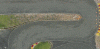TopDown 2D car physics

MrFranz

Member
Hi, I'm quite new here (still learning the basics of Space Rocks) and I'm scouring the forum to find info on 2D car physics.

I came across a 2017 discussion (post: 147991) and a tutorial from @GMWolf but I think I'm doing something wrong, as I get a compiling error even if I copied exactly the code shown in the video.

Does anyone know why I'm getting the error and if there is any other interesting more recent tutorial?

Thanks,

View attachment 43869

Looks like the tutorial was for an older version and the syntax is not working with GMS 2.3.5.589, so I'm experimenting a little bit and below you can see what I came up with.
The car handling is ok but there is no drifting at all and I also need to implement the R gear (which it's harder than I thought).
I tried reading the manual for "physics_apply_local_impulse" but it is maybe outside my current level of understanding, cause I can't make it work.

If any of the good souls in this forum have suggestions it would be much appreciatedGML:
///Vehicle handling

var turn_l = keyboard_check(vk_left);
var turn_r = keyboard_check(vk_right);
var gas = keyboard_check(vk_control);
var breaking = keyboard_check(vk_alt);
var steering = 3;
var acceleration = 0.1
var max_speed = 10;
var ground_friction = 0.01;
image_angle = direction;

//Steering
if speed > 0.2 {
if turn_l { direction += steering; }
if turn_r { direction -= steering; }
}

//Moving
if gas {
if speed < max_speed {
//ADD PHYSICS IMPULSE to allow drifting
}
}

//Breaking
if breaking {
if speed > 0.2 {

}

if speed > 0 {
speed -= ground_friction; }
PROGRESS UPDATE:

So, basically I was trying to mix normal scripting with the built-in physics of GMS and that was basically preventing the car from moving.

Now I've switched to full in-engine physics and updated the script with the physics syntax...and it works. Sort of...

I still can't find a way to kill the sideway motion when the car stops accelerating/turningHere is the code so far:

///Vehicle handling

var turn_l = keyboard_check(vk_left);
var turn_r = keyboard_check(vk_right);
var gas = keyboard_check(vk_control);
var breaking = keyboard_check(vk_alt);
var steering = 2;
var acceleration = 0.1;

//Steering
if phy_speed > 0.1 {
if turn_l { phy_rotation -= steering; }
if turn_r { phy_rotation += steering; }
}

//Moving
if gas {

xpos = lengthdir_x(acceleration, -phy_rotation);
ypos = lengthdir_y(acceleration, -phy_rotation);

physics_apply_impulse(x, y, xpos, ypos);

}

I'll continue to tinker with the code and try to limit the sideway motion. Suggestions will be much appreciated